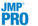Predictive and Specialized Modeling > Naive Bayes > Naive Bayes Platform Options
Publication date: 07/30/2020

#Naive Bayes Platform Options

The Naive Bayes red triangle menu contains the following options:

ROC Curve

Shows or hides the ROC plot. The ROC plot is shown by default. See ROC Curves.

Lift Curve

Shows or hides the lift curve for the model. If you used validation, Lift curve is shown for each of the Training, Validation, and Test sets, if specified.

A lift curve shows how effectively response levels are classified as their fitted probabilities decrease. The fitted probabilities are plotted along the horizontal axis in descending order. The vertical coordinate for a fitted probability is the proportion of correct classifications for that probability or higher, divided by the overall correct classification rate. Use the lift curve to see whether you can correctly classify a large proportion of observations if you select only those with a fitted probability that exceeds a threshold value.

If the response has two levels, the Lift curve plot displays a lift curve for the first level of the response only. If the response has more than two levels, the Lift curve plot displays a sub-outline of the curves for each response level. See Lift Curve in the Partition Models section for more information about lift curves.

Save Predicteds

Saves the predicted classifications to the data table in a column called Naive Predicted <Y, Response>.

Save Prediction Formula

Saves a column called Naive Predicted Formula <Y, Response> to the data table. This column contains the prediction formula for the classifications.

Save Probability Formula

Saves columns to the data table that contain formulas used for classifying each observation. Three groups of columns are saved:

Naive Score <Class>, Sum

For each column that represents a class, this column gives a score formula that measures strength of membership in the given class. In the Naive Score Sum column., these scores are summed across classes. See Saved Probability Formulas.

Naive Prob <Class>

For each class, this column gives a formula for the conditional probability that an observation is in that class. See Saved Probability Formulas.

Naive Predicted Formula <Y, Response>

Gives the formula for the predicted class.

Publish Probability Formula

Creates probability formulas and saves them as formula column scripts in the Formula Depot platform. If a Formula Depot report is not open, this option opens the Formula Depot window. See Formula Depot.

Profiler

Shows or hides an interactive profiler report. Changes in the factor values are reflected in the estimated classification probabilities. See Profiler in Profilers.

Local Data Filter

Shows or hides the local data filter that enables you to filter the data used in a specific report.

Redo

Contains options that enable you to repeat or relaunch the analysis. In platforms that support the feature, the Automatic Recalc option immediately reflects the changes that you make to the data table in the corresponding report window.

Save Script

Contains options that enable you to save a script that reproduces the report to several destinations.

Save By-Group Script

Contains options that enable you to save a script that reproduces the platform report for all levels of a By variable to several destinations. Available only when a By variable is specified in the launch window.